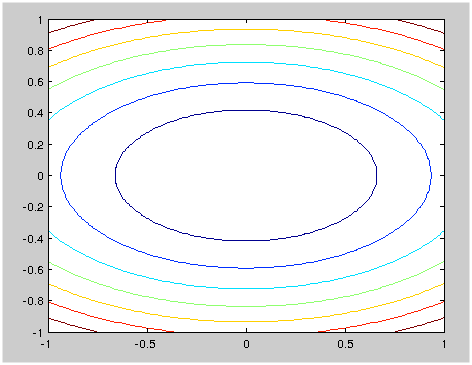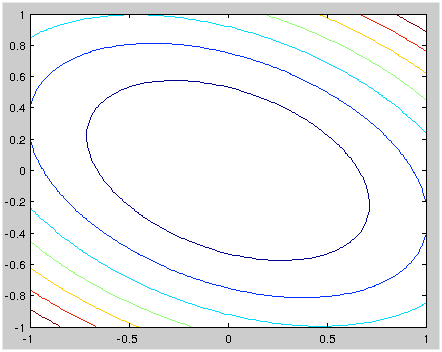## Rotation of ellipse and eigenvectors of quadratic forms

Rotation of ellipse and eigenvectors of quadratic forms

A quaratic form is a polynomial of the form:

\begin{align*} f(x) &= x^TAx - b^T x + c \\ \end{align*}

Where x is a vector. When x is 2D, then this form could be a paraboloid geometrically (try to expand it). For simplicity's sake, take the following as an example (let b = 0):

\begin{align*} f(x) &= \begin{pmatrix} x_1 & x_2 \\ \end{pmatrix} \begin{pmatrix} a & 0 \\ 0 & b \\ \end{pmatrix} \begin{pmatrix} x_1 \\x_2 \end{pmatrix} \\ &= ax_1^2 + bx_2^2 \\ \end{align*}

At each level of f(x), its shape is an ellipse, with the main axes lying on x and y-axis.

The directions of these two main axes of the ellipse are the same as the eigen vectors of A (try calculate these).

Suppose we rotate the ellipse a little, will this still be true?

Let $x = R_\theta u$,

\begin{align*} x &= \begin{pmatrix} \cos \theta & -\sin \theta \\ \sin \theta & \cos \theta \\ \end{pmatrix} u \end{align*}

After some algebra we will have:

\begin{align*} (a\cos^2\theta + b\sin^2 \theta)u^2 + (b\cos^2\theta + a\sin^2 \theta)v^2 + (b\sin 2\theta - a\sin 2\theta)uv = c \end{align*}

This is also a ellipse, and if a = b, as you can see, the rotation would be in vain.

There is a simpler way, though. We can rewrite the original equation as

\begin{align*} f(x) &= x^TAx \\ &= u^TR_\theta^T A R_\theta u \\ &= u^T A_1 u \\ \end{align*}

This is the same as the original in form. Note that every point has been rotated $-\theta$, and indeed if $v$ is an eigenvector of A, then $R_\theta^T v$, or equivalently $R_{-\theta}v$, is an eigenvector of $A_1$, (as I have proved in another post.) , which means the eigenvectors have rotated the same angle as all the points.

This can be visualized with matlab:Rotate a quadratic form by transforming the A matrix (matlab code)
Click to toggle code (may have to try twice)

%%%%%%%%%%%%%%%%%%%%%%%%%%%%%%%%%%%%%%%%%%%%%%%
% In this demonstration I first plot the
% contour of a quadratic form, then
% rotate it by -30 degrees, and plot
% the contour again.
%%%%%%%%%%%%%%%%%%%%%%%%%%%%%%%%%%%%%%%%%%%%%%%
n = 100
A = [2 0; 0 5];
x = linspace(-1, 1, n)
y = linspace(-1, 1, n)
[X, Y] = meshgrid(x, y);
X = X(:); X=X';
Y = Y(:); Y=Y';
u = vertcat(X, Y);

fx = u' * A * u;
fx = diag(fx);
fx = reshape(fx, n, n);
X = reshape(X, n, n);
Y = reshape(Y, n, n);
size(fx)
size(X)
size(Y)
contour(X, Y, fx, 7)

R30 = [cosd(30) -sind(30); sind(30) cosd(30)];
A1 = R30' * A * R30;
fx = u' * A1 * u;
fx = diag(fx);
fx = reshape(fx, n, n);
X = reshape(X, n, n);
Y = reshape(Y, n, n);
size(fx)
size(X)
size(Y)
contour(X, Y, fx, 7)